Question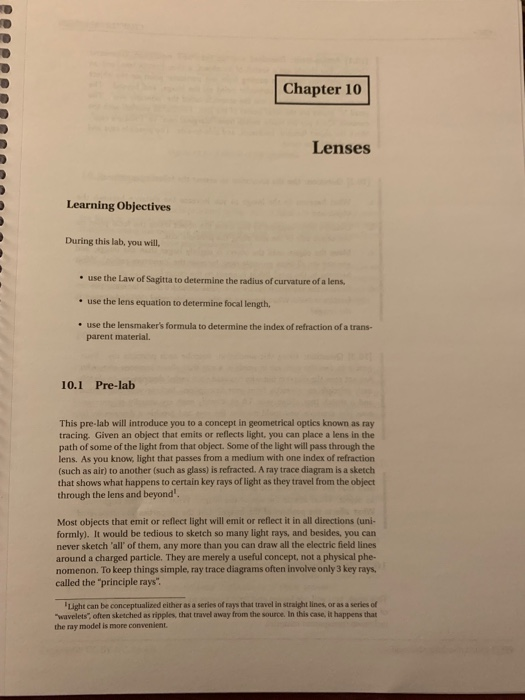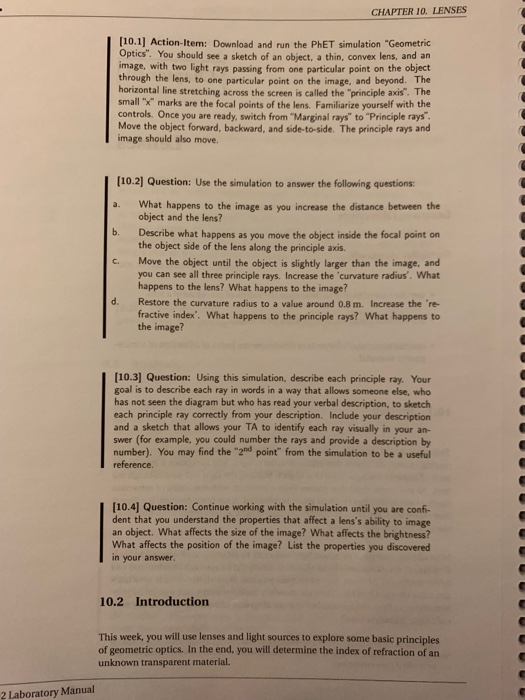Chapter 10 Lenses Learning Objectives During this lab, you will, use the Law of Sagitta to determine the radius of curvature of a lens use the lens equation to determine focal length, . use the lensmaker's formula to determine the index of refraction of a trans- parent material. 10.1 Pre-lab This pre-lab will introduce you to a concept in geometrical optics known as ray tracing. Given an object that emits or reflects light, you can place a lens in the path of some of the light from that object. Some of the light will pass through the lens. As you know, light that passes from a medium with one index of refraction (such as air) to another (such as glass) is refracted. A ray trace diagram is a sketch that shows what happens to certain key rays of light as they travel from the object through the lens and beyond Most objects that emit or reflect light will emit or reflect it in all directions (uni- formly). It would be tedious to sketch so many light rays, and besides, you can never sketch 'all' of them, any more than you can draw all the electric field lines around a charged particle. They are merely a useful concept, not a physical phe- nomenon. To keep things simple, ray trace diagrams often involve only 3 key rays, called the "principle rays Light can be conceptualized either as a series of tays that travel in straight lines, or as a series of "wavelets", often sketched as ripples, that travel away from the source. In this case, it happens that the ray model is more convenient

10.2

a) As the object gets far from the lens, the image becomes smaller and moves towards the lens.

b) The refracted rays diverge as the object moves inside the focal point of the lens. They converge on the object side of the lens and create a bigger virtual image.

c) The lens becomes thinner as the radius of curvature is increased. The image moves further from the lens and gets bigger as the radius of curvature is increased.

d) As the refractive index is increased the principle rays bend closer to the axis of the lens. The image comes closer to the lens and turns smaller.

10.3

Principle ray descriptions:

The principle ray that goes through the lens and parallel to the principle axis goes through the focal point after refraction.

The principle ray that goes through the focal point on the object side of the lens and through the lens refracts out as a parallel ray to the principle axis.

The principle ray that goes through the central point if the lens remains straight after refraction.

10.4

Size of the image is affected by the distance between the lens and the object, refractive index and radius of curvature. If the object is outside of focal point, more the distance, smaller the image. Inside the focal point, more distance means bigger object. Increasing radius of curvature increases image distance, increasing refractive index decreases the distance between the lens and the image.

Brightness of the image is also affected by the distance between the lens and the object, and the size of the lens. More the distance, dimmer the image. Larger the lens brighter the object.

Position of the image is entirely dependent on the object's position. If the object is outside the focal point (object side) the image is going to be on the other side. If the object is inside the focal point of the lens the image is going to be virtual, and on the same side as the object.

#### Earn Coins

Coins can be redeemed for fabulous gifts.

Similar Homework Help Questions
• ### Image Saeen Convex Light Source and Objact Figure 7.1- A sketch of the apparatus run the PhET s...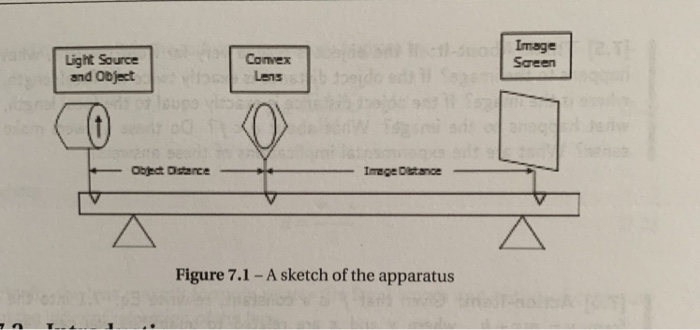7.2?7.3?7.4? Image Saeen Convex Light Source and Objact Figure 7.1- A sketch of the apparatus run the PhET simulation "Geometric 7.1] Action-Item: Download and Optics". You should see a sketch of an object, a thin, convex lens, and an image, with two light rays passing from one particular point on the object through the lens, to one particular point on the image, and beyond. The horizontal line stretching across the screen is called the "principle axis". The small "x" marks...

• ### CONVEX LENSES Optics 173 In this tutorial, use a straightedge to draw lines that are meant...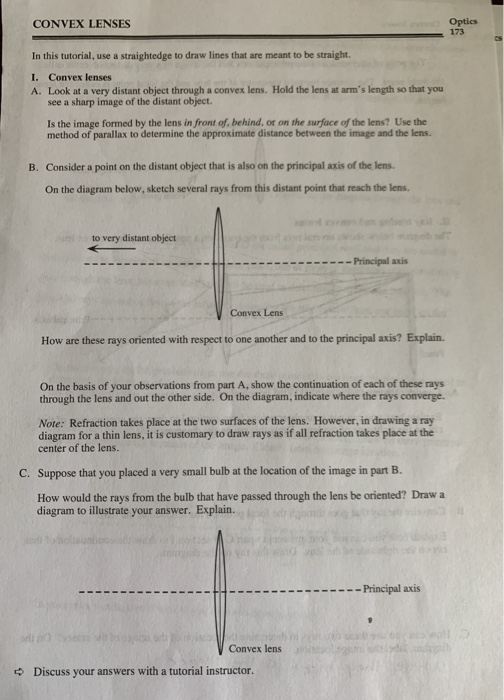CONVEX LENSES Optics 173 In this tutorial, use a straightedge to draw lines that are meant to be straight. I. Convex lenses A. Look at a very distant object through a convex lens. Hold the lens at arm's length so that you see a sharp image of the distant object. Is the image formed by the lens in front of behind, or on the surface of the lens? Use the method of parallax to determine the approximate distance between the...

• ### Name: Lab: Lens Ray Tracing Exercise Physics 182 Date:_ Ray Tracing for Lenses Predict the variou...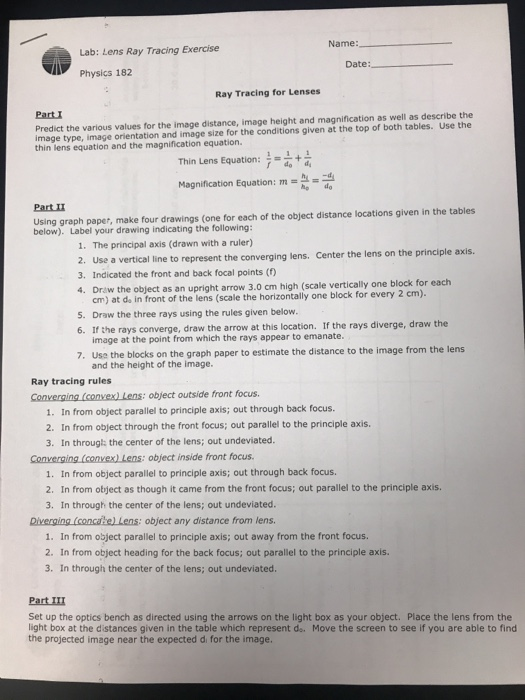Name: Lab: Lens Ray Tracing Exercise Physics 182 Date:_ Ray Tracing for Lenses Predict the various values for the image distance, image height and magnification as well as describe the image type, image orientation and image size for the conditions given at the top of both tables. Use the thin lens equation and the magnification equation. Part Thin Lens Equation: = Magnification Equation: m Parti beton preoah ger ranlonch of the object ditance loatons aven in the tables 1. The...

• ### Optics and Converging & Diverging Lenses 1. What is the relationship between the curvature of a...

Optics and Converging & Diverging Lenses 1. What is the relationship between the curvature of a lens (thick vs thinner converging lenses) and its light-bending power? 2. Focal length: is there a trend in the image distances vis a vis focal lengths as the object distance gets larger? What happens to the thin lens formula when object distance is very large? 3. In the compound lens formula, 1/fcomp = 1/f1 + 1/f2, why does a longer focal lens will not...

• ### Problem 9: Go to the PhET website and run the geometrics optics simulation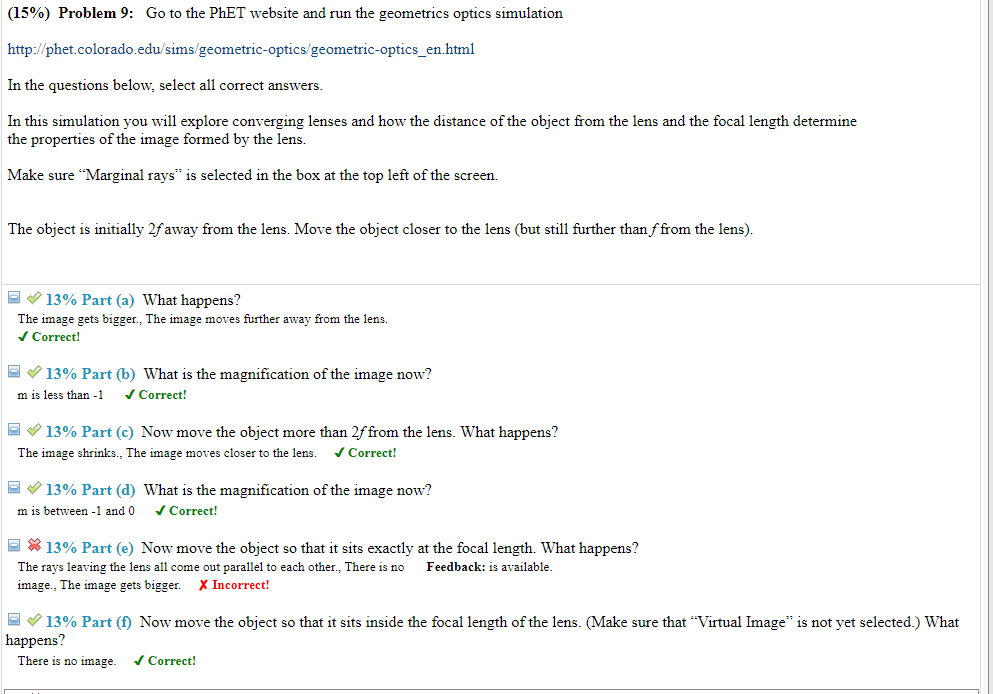Problem 9: Go to the PhET website and run the geometrics optics simulationhttp://phet.colorado.edu/sims/geometric-optics/geometric-optics_en.htmlIn the questions below, select all correct answers.In this simulation you will explore converging lenses and how the distance of the object from the lens and the focal length determine the properties of the image formed by the lens.Make sure "Marginal rays" is selected in the box at the top left of the screen.The object is initially 2 f away from the lens. Move the object closer to...

• ### 3. Optics problem set: a) [6 pts) An incident light ray in air strikes a glass...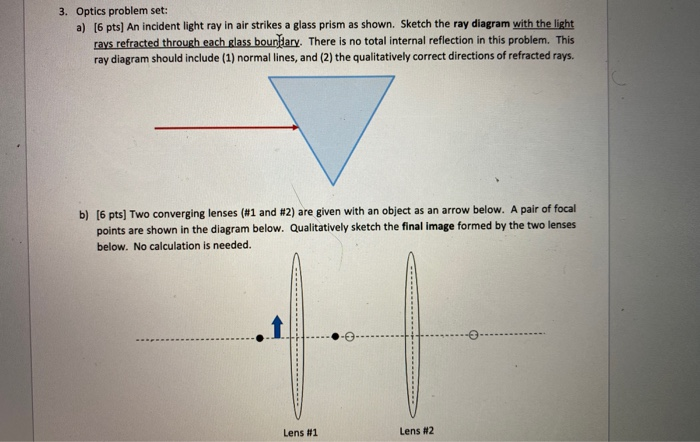3. Optics problem set: a) [6 pts) An incident light ray in air strikes a glass prism as shown. Sketch the ray diagram with the light rays refracted through each glass boundary. There is no total internal reflection in this problem. This ray diagram should include (1) normal lines, and (2) the qualitatively correct directions of refracted rays. b) [6 pts) Two converging lenses (#1 and #2) are given with an object as an arrow below. A pair of focal...

• ### this is what they gave us 4. Use the focal length you found in (3), complete...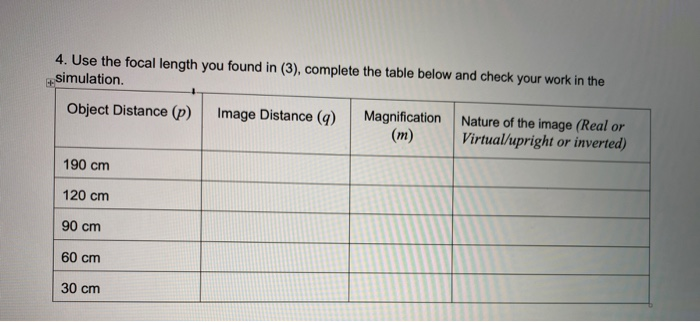this is what they gave us 4. Use the focal length you found in (3), complete the table below and check your work in the simulation. Object Distance (p) Image Distance (9) Magnification Nature of the image (Real or (m) Virtual/upright or inverted) 190 cm 120 cm 90 cm 60 cm 30 cm Online Lab #9 3. Verify the focal length of the lens: a. Set the refractive index (n) to 1.5 and the radius of curvature (R) to 0.6...

• ### corrective Lenses (@) Spectacles are often coated to reduce reflection. The plastic of the lens has...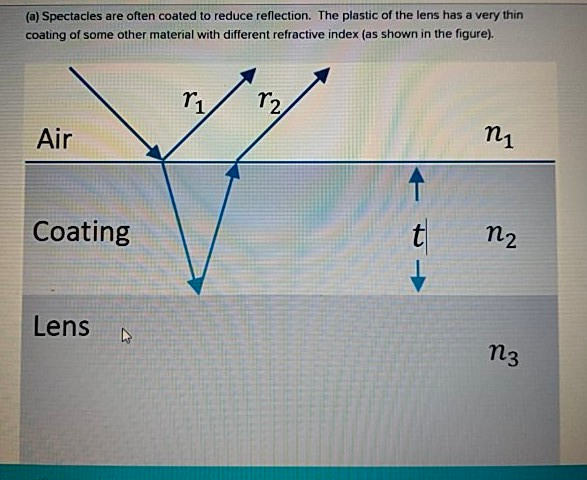corrective Lenses (@) Spectacles are often coated to reduce reflection. The plastic of the lens has a very thin coating of some other material with different refractive index (as shown in the figure). 11 T2 Air ni Coating TLV n2 Lens n3 Figure - cross section showing three parallel layers of material (air, coating and the lens), with a light ray incident on the coating. The ray is partially reflected at the air/coating interface; the reflected ray Ti is shown....

• ### PRE-LAB INVESTIGATION: Review the use of ray diagrams to help you to determine how and where...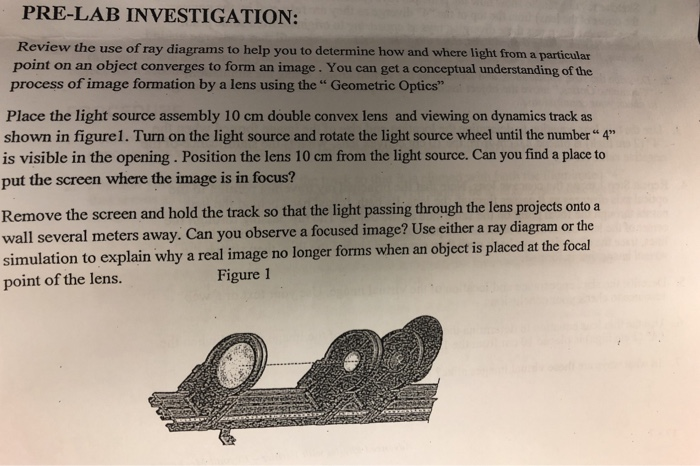PRE-LAB INVESTIGATION: Review the use of ray diagrams to help you to determine how and where light from a particular point on an object converges to form an image. You can get a conceptual understanding of the process of image formation by a lens using the " Geometric Optics" Place the light source assembly 10 cm double convex lens and viewing on dynamics track as shown in figurel. Turn on the light source and rotate the light source wheel until...

• ### 4:58 4 LTE Object POST-LAB Questions and Image Distance - Thin Lenses NAME JAIME 1. The...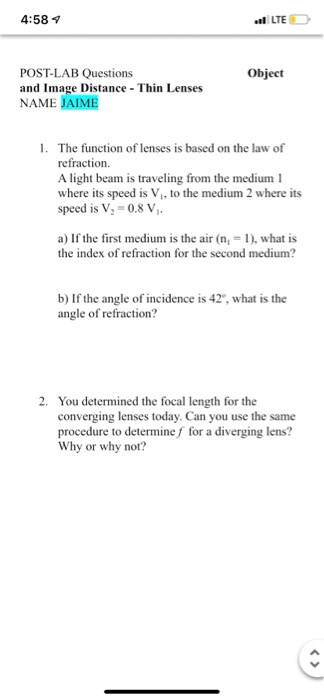4:58 4 LTE Object POST-LAB Questions and Image Distance - Thin Lenses NAME JAIME 1. The function of lenses is based on the law of refraction A light beam is traveling from the medium 1 where its speed is V., to the medium 2 where its speed is V2 = 0.8 V, a) If the first medium is the air (n = 1), what is the index of refraction for the second medium? b) If the angle of incidence is...